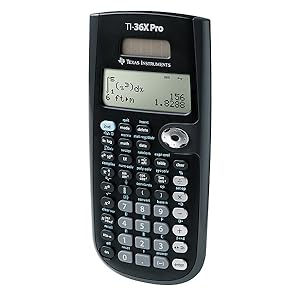# Texas Instruments TI-36X Pro Engineering,Scientific Calculator

• Ideal for curricula in which graphing technology may not be permitted.
• MultiView display shows multiple calculations at the same time on screen.
• MathPrint shows math expressions, symbols and stacked fractions as they appear in textbooks
• Ideal for high school through college: Algebra 1 & 2, Geometry, Trigonometry, Statistics, Calculus, Biology, etc.
• Convert fractions, decimals and terms including Pi into alternate representations.
• Select degrees/radians, floating/fix, number format modes.

Units Sold: 0Texas Instruments TI-36X Pro Engineering,Scientific Calculator

\$44.99

### Texas Instruments TI-36X Pro

One of the most advanced of many, this Texas Instruments calculator features functional MathPrint capabilities. On the Texas Instruments TI-36X Pro users can utilize MathPrint for viewing calculations in math notations, including the answers to fractions, square roots, and pi. Extra enhanced functionality in math makes the Texas Instruments TI-36X ideal for science and engineering courses, as well as classes where graphing technologies may not be allowed. For a scientific calculator, this product comes packed full of features such as the easy to read LCD display, quick reset, and hard plastic smooth coated keys. With a four-line LCD display as well as three solvers for numeric equation, linear equations, and polynomial operations, this Texas Instruments calculator is highly flexible and functional. With numeric derivatives and integral options for real functions, the Texas Instruments TI-36X has a plethora of engineering and scientific notations. On the Texas Instrument calculator, consumers can enjoy three memory options for storing intermediate results. Being a scientific calculator, this device is also helpful for trigonometry, advanced algebra, computer sciences, and statistics.

##Advanced, four-line scientific calculator, with higher-level math and science functionality, that is ideal for computer science and engineering courses in which graphing technology may not be permitted.

Ideal for students at every level, from junior high school algebra to college calculus, the Texas Instruments TI36 packs a lot of punch in a small, inexpensive unit. This solar-powered calculator combines features for statistical analysis, Boolean logic operations, and unit conversions in one sleek package.

Math students will appreciate TI36X’s ability to perform fractional equations and calculate angle units (radians, grads, degrees) when performing trigonometric functions. Everyone will find the calculator’s English/metric conversions helpful.

The TI36Xs single-line LCD is a little difficult to read when the unit is lying on a desk, and users in need of graphing functions will have to look elsewhere. Overall, however, for a calculator in this price range, the Texas Instruments TI-36X Pro provides enough power for most high-level math and science needs.

## Specification: Texas Instruments TI-36X Pro Engineering,Scientific Calculator

Brand Texas Instruments 0666672977165 36PRO/TBL/1L1/A 3 3/8 x 7 1/4 English 30 days

## User Reviews

0.0 out of 5
0
0
0
0
0

There are no reviews yet.• Total (0)
error: